# Dupin indicatrix

(diff) ← Older revision | Latest revision (diff) | Newer revision → (diff)

curvature indicatrix

A plane curve illustrating the normal curvatures of a surface at a point of this surface. The Dupin indicatrix lies in the tangent plane to the surfaceat the pointand there it is described by the radius vectorof length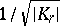, where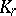is the normal curvature ofatin the direction. Let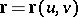be a parametrization ofin a neighbourhood of. One introduces a coordinate system on the tangent plane toat, takingas the coordinate origin, and the vectors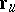and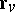as the basis vectors of this coordinate system. The equation of the Dupin indicatrix will then bewhereandare the coordinates of a point on the Dupin indicatrix, and,andare the coefficients of the second fundamental form ofcalculated at. The Dupin indicatrix is: a) an ellipse ifis an elliptic point (a circle ifis an umbilical point); b) a pair of conjugate hyperbolas ifis a hyperbolic point; and c) a pair of parallel straight lines ifis a parabolic point. The curve is named after Ch. Dupin (1813), who was the first to use this curve in the study of surfaces.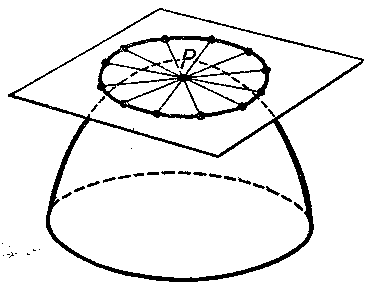Figure: d034180a

How to Cite This Entry:
Dupin indicatrix. Encyclopedia of Mathematics. URL: http://encyclopediaofmath.org/index.php?title=Dupin_indicatrix&oldid=13694
This article was adapted from an original article by E.V. Shikin (originator), which appeared in Encyclopedia of Mathematics - ISBN 1402006098. See original article